# Graph the result of an array containing the data

Get the data from an array, a CSV, a JSON and draw it !

``````\$graph = new Domframework\Graph ();
// For CSV:
\$graph->data->csv ("\"\",Jan,Feb,Mar,Apr,May\n".
"Prod,200,210,NO,230,240\n".
"Return,5,4,3,2,1");
// For JSON:
\$graph->data->json (json_encode (array (array ("T1","1","2","3","4"),
array ("T2","5","6","7","8"))));
// For indexed array:
\$graph->data->arrayIndexed (array (array ("T1","1","2","3","4"),
array ("T2","5","6","7","8")));
// For associated array:
\$graph->data->arrayAssociative (array (array ("t1"=>"11","t2"=>"12","t3"=>"13"),
array ("t1"=>"21","t2"=>"22","t3"=>"23"),
array ("t1"=>"31","t2"=>"32","t3"=>"33")));

\$graph->drawImage();``````

## The styles

The series can be drawn by different styles : line, linePoints, points.

``````\$graph = new Domframework\Graph ();
\$graph->data->csv ("\"\",Jan,Feb,Mar,Apr,May\n".
"Prod,200,210,NO,230,240\n".
"Return,5,4,3,2,1")
->titlesOnFirstLine (true)
->titlesOnFirstColumn (true)
->horizontalData (true);
\$graph->series->serie ("Return")->axisYsecondary(true);
\$graph->drawImage ();``````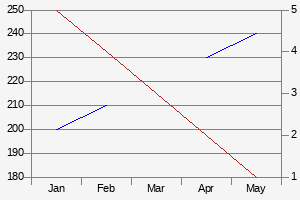``````\$graph = new Domframework\Graph ();
\$graph->data->csv ("\"\",Jan,Feb,Mar,Apr,May\n".
"Prod,200,210,NO,230,240\n".
"Return,5,4,3,2,1")
->titlesOnFirstLine (true)
->titlesOnFirstColumn (true)
->horizontalData (true);
\$graph->series->serie ("Return")->axisYsecondary(true);
\$graph->style ("linePoints");
\$graph->drawImage ();``````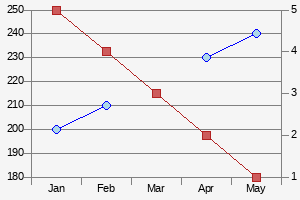``````\$graph = new Domframework\Graph ();
\$graph->data->csv ("\"\",Jan,Feb,Mar,Apr,May\n".
"Prod,200,210,NO,230,240\n".
"Return,5,4,3,2,1")
->titlesOnFirstLine (true)
->titlesOnFirstColumn (true)
->horizontalData (true);
\$graph->series->serie ("Return")->axisYsecondary(true);
\$graph->style ("points");
\$graph->drawImage ();``````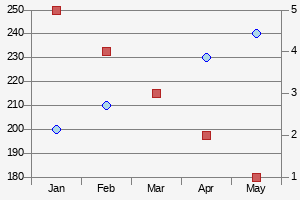## The integrated objects

The graph object carry some objects specialized in functions:

• The graph object manage all the other ones. It allow to define the default style, the height and width of the drawn graph, the title position
``````\$graph->style ("linePoints")
->width (300)
->height (200);``````
• The data object: manage the input of the data (from array, CSV, JSON). Allow to manage if there is a title on first line or column with autodetection. It also permit to say if the data are stored horizontally or vertically
• The title object: manage the title of the graph, with font, size, color and text.
``````\$graph->title->text ("The SUPER Title")
->color ("orange")
->fontsize (10)
->fontfile ("/usr/share/fonts/truetype/ttf-bitstream-vera/VeraBd.ttf");``````

Example :

``````\$graph = new Domframework\Graph ();
\$graph->title->text ("The SUPER Title")
->color ("orange")
->fontsize (10)
->fontfile ("/usr/share/fonts/truetype/ttf-bitstream-vera/VeraBd.ttf");
\$graph->data->csv ("\"\",Jan,Feb,Mar,Apr,May\n".
"Prod,200,210,NO,230,240\n".
"Return,5,4,3,2,1")
->titlesOnFirstLine (true)
->titlesOnFirstColumn (true)
->horizontalData (true);
\$graph->series->serie ("Return")->axisYsecondary(true);
\$graph->style ("points");
\$graph->drawImage ();``````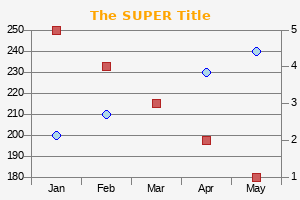• The legend object: manage the display of a legend, with samples, series names, background-color, font and font size.
``\$graph->legend->show (true);``

Example :

``````\$graph = new Domframework\Graph ();
\$graph->title->text ("Display the legend")
->fontsize (10)
->fontfile ("/usr/share/fonts/truetype/ttf-bitstream-vera/VeraBd.ttf");
\$graph->data->csv ("\"\",Jan,Feb,Mar,Apr,May\n".
"Prod,200,210,NO,230,240\n".
"Return,5,4,3,2,1")
->titlesOnFirstLine (true)
->titlesOnFirstColumn (true)
->horizontalData (true);
\$graph->series->serie ("Return")->axisYsecondary(true);
\$graph->style ("linePoints");
\$graph->legend->show (true);
\$graph->drawImage ();``````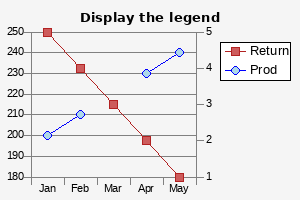• The series object manage the data series. It is an abstraction class.
• The serie object, provided by the \$graph->series->serie ("NAME"), allow to manage the serie. The serie can be drawn on the secondary axis, can have a maximum or minimum value, can have a style different of the general one defined in graph object. It allow to define if the provided data are numerical or labeled
``````\$graph->series->serie ("Return")->style ("line")
->axisYsecondary(true);``````

Example :

``````\$graph = new Domframework\Graph ();
\$graph->style ("linePoints");
\$graph->title->text ("Default style in linePoints, one in line")
->fontsize (10)
->fontfile ("/usr/share/fonts/truetype/ttf-bitstream-vera/VeraBd.ttf");
\$graph->data->csv ("\"\",Jan,Feb,Mar,Apr,May\n".
"Prod,200,210,NO,230,240\n".
"Return,5,4,3,2,1")
->titlesOnFirstLine (true)
->titlesOnFirstColumn (true)
->horizontalData (true);
\$graph->series->serie ("Return")->axisYsecondary(true)->style("line");
\$graph->drawImage ();``````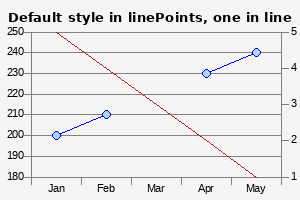• The axis object allow to manage the colors, fonts (type and size). The objects defined can be used by \$graph->axisX, \$graph->axisY1 or \$graph->axisY2
``````\$graph->axisY1->min (10)->max (300)->numerical (true);
\$graph->axisY2->min (-10)->max (10)->numerical (true);
\$graph->axisY1->gridColor ("blue");
\$graph->axisY2->gridColor ("blue");
\$graph->axisX->gridColor ("blue");``````

Example :

``````\$graph = new Domframework\Graph ();
\$graph->title->text ("Grids in blue color")
->fontsize (10)
->fontfile ("/usr/share/fonts/truetype/ttf-bitstream-vera/VeraBd.ttf");
\$graph->data->csv ("\"\",Jan,Feb,Mar,Apr,May\n".
"Prod,200,210,NO,230,240\n".
"Return,5,4,3,2,1")
->titlesOnFirstLine (true)
->titlesOnFirstColumn (true)
->horizontalData (true);
\$graph->series->serie ("Return")->axisYsecondary(true);
\$graph->style ("points");
\$graph->axisY1->min (10)->max (300)->numerical (true);
\$graph->axisY2->min (-10)->max (10)->numerical (true);
\$graph->axisY1->gridColor ("blue");
\$graph->axisY2->gridColor ("blue");
\$graph->axisX->gridColor ("blue");
\$graph->drawImage ();``````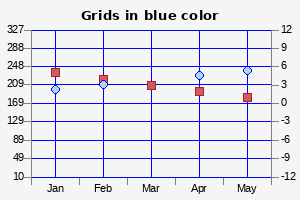## Class Domframework\Graph

Namespace Domframework

### Description

```/**
This class allow to generate an image which is a graphic. A graphic takes
an array of values and draw the lines/histo... like a spreadsheet
graph methods are :
->height (\$height) or ->width (\$width) The heigh/width of the graph
```

### Properties

##### public \$axisX;
```/**
The X axis object
```
##### public \$axisY1;
```/**
The main Y axis object
```
##### public \$axisY2;
```/**
The optional secondary Y axis object
```
```/**
The data object
```
##### public \$legend;
```/**
The legend object
```
##### public \$series;
```/**
The series object
```
##### public \$title;
```/**
The graph title object
```

### Methods

##### public function __construct ()
```/**
Constructor : create the objects
```

##### public function bgcolor ( \$bgcolor=null)
```/**
Set the background-color of the graph if the parameter is provided.
Get the background-color of the graph if the parameter is not provided
`@param string|null \$bgcolor` The background-color of the graph
```

##### public function drawBase64 ()
```/**
Return the image coded in base64
`@return` string The base64 string
```

##### public function drawImage ()
```/**
Draw the graph to the screen with the previous defined parameters
```

##### public function height ( \$height=null)
```/**
Set the height of the graph if the parameter is provided.
Get the height of the graph if the parameter is not provided
`@param integer|null \$height` The height of the graph
```

##### public function style ( \$style=null)
```/**
Set the default style of the graph if the parameter is provided.
Get the default style of the graph if the parameter is not provided
`@param string|null \$style` The style of the graph
```

##### public function titlePosition ( \$titlePosition=null)
```/**
Set the title position of the graph if the parameter is provided.
Get the title position of the graph if the parameter is not provided
`@param string|null \$titlePosition` The title position of the graph
```

##### public function width ( \$width=null)
```/**
Set the width of the graph if the parameter is provided.
Get the width of the graph if the parameter is not provided
`@param integer|null \$width` The width of the graph
```